# Pentagon problem

 Importance: High ✭✭✭
 Author(s): Nesetril, Jaroslav
 Subject: Graph Theory » Coloring » » Homomorphisms
 Keywords: cubic homomorphism
 Posted by: Robert Samal on: March 24th, 2007
Question   Letbe a 3-regular graph that contains no cycle of length shorter than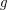. Is it true that for large enough~there is a homomorphism?

This question was asked by Nesetril at numerous problem sessions (and also appears as [N]). By Brook's theorem any triangle-free cubic graph is 3-colorable. Does a stronger assumption on girth of the graph imply stronger coloring properties?

This problem is motivated by complexity considerations [GHN] and also by exploration of density of the homomorphism order: We write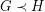if there is a homomorphism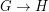but there is no homomorphism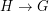. It is known that wheneverholds and~is not bipartite, there is a graph~satisfying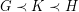. A negative solution to the Pentagon problem would have the following density consequence: for each cubic graph~for which~holds, there exists a cubic graph~satisfying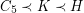(see [N]).

If we replacedin the statement of the problem by a longer odd cycle, we would get a stronger statement. It is known that no such strenghthening is true. This was proved by Kostochka, Nesetril, and Smolikova [KNS] for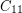(hence for allwith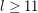), by Wanless and Wormald [WW] for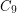, and recently by Hatami [H] for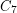. Each of these results uses probabilistic arguments (random regular graphs), no constructive proof is known.

Haggkvist and Hell [HH] proved that for every integer~there is a graph~with odd girth at least~(that is,does not contain odd cycle of length less than~) such that every cubic graph of odd girth at least~maps homomorphically to~. Here, the graph~may have large degrees. This leads to a weaker version of the Pentagon problem:

Question   Is it true that for everythere exists a cubic graph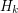of girth~and an integer~such that every cubic graph of girth at least~maps homomorphically to~?

A particular question in this direction: does a high-girth cubic graph map to the Petersen graph?

As an approach to this, we mention a result of DeVos and Samal [DS]: a cubic graph of girth at least~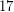admits a homomorphism to the Clebsch graph. In context of the Pentagon problem, the following reformulation is particularly appealing: If~is a cubic graph of girth at least~, then there is a cut-continuous mapping from~to~; that is, there is a mappingsuch that for any cut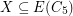the preimage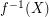is a cut. (Here by cut we mean the edge-set of a spanning bipartite subgraph. A more thorough exposition of cut-continuous mappings can be found in~[DNR].)

## Bibliography

[DNR] Matt DeVos, Jaroslav Nesetril, and Andre Raspaud, On edge-maps whose inverse preverses flows and tensions, Graph Theory in Paris: Proceedings of a Conference in Memory of Claude Berge, Birkhäuser 2006.

[DS] Matt DeVos and Robert Samal, High girth cubic graphs map to the Clebsch graph

[GHN] Anna Galluccio, Pavol Hell, and Jaroslav Nesetril, The complexity of-colouring of bounded degree graphs, Discrete Math. 222 (2000), no.~1-3, 101--109, MathSciNet

[HH] Roland Haggkvist and Pavol Hell, Universality of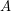-mote graphs, European J. Combin. 14 (1993), no.~1, 23--27.

[H] Hamed Hatami, Random cubic graphs are not homomorphic to the cycle of size~7, J. Combin. Theory Ser. B 93 (2005), no.~2, 319--325, MathSciNet

[KNS] Alexandr~V. Kostochka, Jaroslav Nesetril, and Petra Smolikova, Colorings and homomorphisms of degenerate and bounded degree graphs, Discrete Math. 233 (2001), no.~1-3, 257--276, Fifth Czech-Slovak International Symposium on Combinatorics, Graph Theory, Algorithms and Applications, (Prague, 1998), MathSciNet

*[N] Jaroslav Nesetril, Aspects of structural combinatorics (graph homomorphisms and their use), Taiwanese J. Math. 3 (1999), no.~4, 381--423, MathSciNet

[WW] I.M. Wanless and N.C. Wormald, Regular graphs with no homomorphisms onto cycles, J. Combin. Theory Ser. B 82 (2001), no.~1, 155--160, MathSciNet

* indicates original appearance(s) of problem.